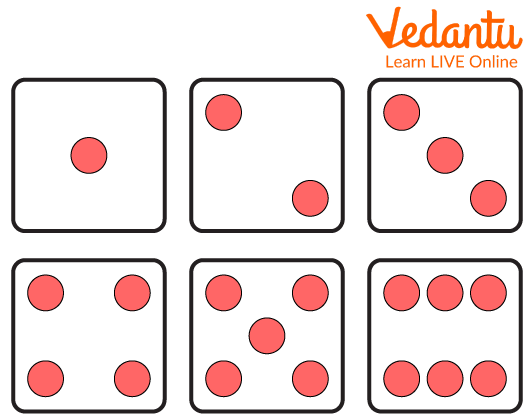Courses
Courses for Kids
Free study material
Free LIVE classes
More

# Sample Space of Two DiceLIVE
Join Vedantu’s FREE Mastercalss

## Introduction to Probability

Probability is also known as a possibility of an event occurring. The probability is never negative and is never more than 1. Some of the real-life situations where probability is used are throwing dice, tossing coins, picking out students from a class, and many more. Probability has long been used in mathematics to approximate how likely events are to occur. Essentially, the probability is the degree to which something is predicted to occur.

## What is Dice?

Dice is a tiny block with one to six marks or tints on its boundary that is used in games to generate a random number. Dice are tiny, tossable blocks having a visible border that can stop in the figures shown.

When thrown or rolled, the die comes to a halt and displays a random number from one to six on its upper side, with the occurrence of each event being equally likely. The dice drawn are used for playing board games as a fun way to relax with family and friends.Possible Outcomes in a Dice

## What is Sample Space?

A sample space is a collection of possible outcomes from a random experiment. The sign "S" represents the sample space. The events of an experiment are a subset of the possible outcomes. A sample region may have a range of results depending on the investigation. It is termed a discrete or finite sample space if it has a finite number of outcomes. Curly brackets "{ }" contain the sample spaces for a random experiment.

## Different Scenarios to Calculate Dice Probabilities

• One dice is thrown- The likelihood of a certain integer happening with one dice is the simplest and most straightforward situation of dice probabilities. Dice shows six possible outcomes. So, the result obtained will be given as: $P\left( A \right) = \dfrac{\text{no of the outcome of A}}{\text{no of total outcomes}}$

• Two dice are thrown - The likelihood of receiving two 6s by tossing two dice is a rare occurrence as the outcome of one die is independent of the outcome of the other dice. The rule of probability applied in such situations states that separate probabilities must be multiplied together to achieve the outcome. As a result, the formula for this is,

• Probability of both $=$ probability of first $\times$ probability of the second

• Questions like two or more than two dice are thrown simultaneously to find the probability of getting a number can be done using the above-mentioned formula.

• The total number from two or more dice - If one wants to know the possibility of receiving a specific sum obtained by rolling two or more dice, one must use the basic rule of probability which is

• Probability = the number of desired results divided by the total number of results.

## Sample Questions

1. A dice has how many faces or sides?

a. 4

b. 5

c. 6

d. 8

Ans: 6

Explanation: A dice is a cuboid that has 6 faces or sides in it.

2. How many possible outcomes would be there if two dice are thrown?

a. 6

b. 12

c. 36

d. 2

Ans: 36

Explanation: One dice has 6 possible outcomes. So, if two dice are rolled then we need to multiply 6 two times which will result in 36. The total number of outcomes in a simultaneous throw of two dice will be 36.

3. Possible outcomes that will come in an experiment are called

a. Sample space

b. Probability

c. Possibility

d. Luck

Ans: Sample space

## Conclusion

Dice is a six-faced three-dimensional object which is used to play board games. When a dice is thrown there are different probabilities of getting a particular result which can be calculated by a probability formula. Sample space is all the possible outcomes that we can get in a particular situation and is useful in finding out the probability of large and complex sample space.

Last updated date: 25th Sep 2023
Total views: 69.6k
Views today: 1.69k

## FAQs on Sample Space of Two Dice

1. Is there a probability of getting a number on a dice more than one?

The probability is never more than 1 or less than 0. So, the probability of getting a digit on a dice is 1 as the number of outcomes is 6, as well as the number we can get on a digit, is also 6.

2. What is the shape of the dice?

The dice is cuboidal in shape with 6 faces having 6 digits printed on it from 1 to 6.

3. What would be the sample space if two coins are tossed?

The sample space of two coins being tossed will be {HH, TT, HT, TH}.

4. Will tossing coins give fair results?

Yes, tossing the coin will give fair results as both the head and tail side of the coin would have a 50-50 chance to appear after the toss.

5. Probability cannot be used in daily life. True or false?

Probability can be used in our daily lives. It is used to predict the nature of the weather conditions, online shopping, online gaming, to make strategies in a game, and is used in many other things too.